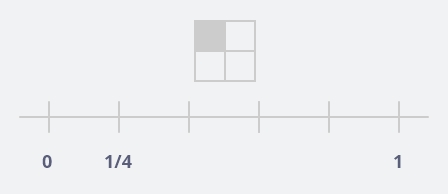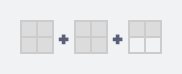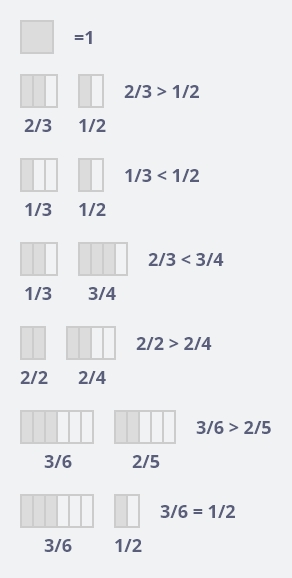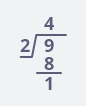Got Feedback?

Found a bug? Have a suggestion? Fill the form below and we'll take a look!

X

# Fraction Calculator

Fraction calculator is a smart tool that is frequently used for tackling the problem related to any proper, improper and mixed fraction. It has capability to shows all the ongoing steps of addition, subtraction, multiplication and division.
This calculator can solve any assign task within seconds. It is reliable and handy online tool. It provides helping hand in measurement.
It will reduce the fraction to it simplest form, that’s make out calculation more simple and understandable. Fraction calculator is widely used in economy, for example;
1- Property division
2- Research
3- Medical field
4- Statistics
5- Cooking/ hotel industry(measuring ingredient in recipes)
6- Time
7- Petrol pumps

## What is Fraction?

Fraction shows how many equal part of a whole and total amount. A fraction is a part of a whole. It represents one or more equal parts of a whole object.

Example: $$\frac{1}{4}$$

Here 1 is a numerator; shows how many of the total equal parts. 4 is the denominator; shows how many total equal parts are in the whole. $$\frac{1}{4}$$ is fraction bar or division bar.

Pictorial Representation:Fraction can have different values depending on a size or amount of the whole. When the numerator and denominator are same number, the fraction will have the same 1, or the whole.
A fraction is also a part of a set.Three fourth $$\frac{3}{4}$$ of the boxes are filled.

## Types of Fractions

1- Proper fraction
2- Improper fraction
3- Mixed fraction

Proper Fraction: A fraction in which the numerator is less than the denominator is called proper fraction. For example, $$\frac{2}{8}$$ here $$2 < 8$$

Improper Fraction: A fraction in which the numerator is greater than or equal to the denominator is called an improper fraction. For example, $$\frac{5}{3}$$ here $$5 > 3$$ and $$\frac{4}{4}$$ here $$4 = 4$$

Mixed Fraction: A mixed fraction is a sum of a natural number and a proper fraction. For example, $$1 + 1 + \frac{1}{2} => 2 + \frac{1}{2} => 2\frac{1}{2}$$## Unit Fraction

A fraction which has 1 as the numerator is a unit fraction.
Example: $$\frac{2}{3}$$, $$\frac{1}{7}$$, $$\frac{1}{12}$$

## Like and unlike fraction

“Like and unlike fraction depend on denominator”

Like fraction:
Fractions with the same denominators are called like fractions. For example, $$\frac{1}{4}$$, $$\frac{3}{4}$$

Unlike fraction:
Fractions with different denominators. For example, $$\frac{5}{8}$$, $$\frac{5}{3}$$, $$\frac{2}{7}$$

## Importance of fraction / Why are fractions important?

Fractions are important because on the basis of it, we can assess what portion of a whole we required, there are few fields where fraction play vital role.
1- Medical field
2- Cooking(measuring ingredients in recipes)
3- Research
4- Statistics(probability)
5- Data representation in mathematics
6- Property division
7- Understanding of an equation( in understanding of an equations in order to proper understanding of equation, we first have a grip on fraction)
$$F = \frac{G m_1 m_2}{r^2}$$
$$K.E = \frac{1}{2}mv^2$$
$$V = \frac{4}{3}πr^3$$
$$A = \frac{1}{2}bh$$
8- Petrol pumps (rates, like 249 $$\frac{9}{10}$$ or RS 148 $$\frac{9}{10}$$)
9- Price list
10- Time (8:45 also means quarter to nine)

## A general example

Q: if a pizza has 8 pieces in it and you give 1 piece away, how many pieces of pizza do you have left?
Answer: let’s make this into a fraction if a fraction is written as $$\frac{a}{b}$$, then this would be written as $$\frac{7}{8}$$ in fraction.

## Comparing fractionQ: Which fraction is greater? $$\frac{2}{5}$$ or $$\frac{6}{5}$$
Solution: As denominator is same so compare only numerator. Therefore; $$\frac{2}{5} < \frac{6}{5}$$

Q: Which fraction is greater? $$\frac{5}{6}$$ or $$\frac{5}{7}$$
Solution: As denominators are different if numerator are same then lesser denominator fraction is the greater one. Therefore; $$\frac{5}{6} > \frac{5}{7}$$ as $$0.83 > 0.7$$

Q: Which fraction is greater? $$\frac{3}{4}$$ or $$\frac{2}{3}$$

Solution: Numerator and denominator are not same, so follow these methods:

(a) Convert each fraction into decimal.

(b) Comparing denominator and then numerator.

(c) Cross multiply

So, $$\frac{3}{4}$$, $$\frac{2}{3}$$ means $$9 > 8$$ hence, $$\frac{3}{4} > \frac{2}{3}$$

Let’s verify the solution $$\frac{3}{4} > \frac{2}{3}$$, its fraction values will be $$0.75 > 0.56$$

Q: Which fraction is greater? $$\frac{4}{9}$$ or $$\frac{5}{7}$$

So, $$\frac{4}{9}$$, $$\frac{5}{7}$$ means $$28 < 45$$ hence, $$\frac{4}{9} < \frac{5}{7}$$

Let’s verify the solution $$\frac{4}{9} < \frac{5}{7} => \frac{4 × 7}{9 × 7} < \frac{5 × 9}{7 × 9} => \frac{28}{63} < \frac{45}{63}$$

## Fraction Tricks

Let’s take example of $$\frac{4}{5}$$, $$\frac{2}{3}$$, $$\frac{5}{6}$$

Solution:

Let’s take LCM of all denominator 5, 3, 6:

 2 5, 3, 6 3 5, 3, 3 5 5, 1, 1 1, 1, 1

Now $$2 × 3 × 5 = 30$$, make all denominator to 30 by multiplying any number to both numerator and denominator.

$$\dfrac{4 × 6}{5 × 6}$$, $$\dfrac{2 × 10}{3 × 10}$$, $$\dfrac{5 × 5}{6 × 5}$$

$$\dfrac{24}{30}$$, $$\dfrac{20}{30}$$, $$\dfrac{25}{30}$$

$$\dfrac{20}{30} < \dfrac{24}{30} < \dfrac{25}{30}$$ Here all denominator are same now we can consider numerator only.
Hence, $$\dfrac{2}{3} < \dfrac{4}{5} < \dfrac{5}{6}$$

## Changing an improper fraction into a mixed number

Convert $$\frac{9}{2}$$ into a mixed number

Solution:

$$Mixed number = quotient + \dfrac{remainder}{division}$$Its mean, $$\frac{9}{2} = 4\frac{1}{2}$$

## Changing a mixed number into an improper fraction

Q: Convert $$5\frac{3}{9}$$ into an improper fraction.

Solution:

$$\frac{(Natural Number × Denominator) + Numerator}{Denominator} => \frac{(5 × 9) + 3}{9} => \frac{45 + 3}{9} => \frac{48}{9}$$

## FAQS

Q1: What are fractions?

Answer: Fractions are piece of whole numbers.

Q2: When will be used fraction?

Answer: Fractions are part and parcel of our daily life transaction as it is everywhere from one form to another.

Q3: How do we convert from a fraction to a decimal number?

Q4: In what grade will I used fraction?

Answer: We are using fraction off and on. As we have been using fraction since out childhood period, when we divided our sweets among out friends.

Q5: Why do we have to reduce fraction?

Answer: This help mathematician to easily interpret data and can also help to avoid confusion when numbers and equation became large and complex.

Q6: What does the parts of a fraction tell about it numerator and denominator?

Answer: The numerator represents how many parts are being considered and the denominator represents the number of equal parts in a whole.

Q7: What is part of whole?

Answer: Part of a whole one is known as a fraction.

Q8: How many quarters make a whole?

Answer: Four quarters makes a whole.

Q9: How many one half are present in a whole?

Q10: What do you understand by “a part of a whole number”?

Answer: A fraction is a number that stands for part of something.

Q11: What types of a jobs use fractions?

Answer: Almost every business. Where tax calculation involve, tips calculation, interest rates uses fractions, banks, restaurants profits. From department stores to the supermarkets all uses percentage where one form to another fraction is used.

Q12: How many fractions used in sports?

Answer: On the basis of fractional change defeat and victory is decided. We use fractions to represent the half-time during any sports.

Q13: Who invented fraction?

Q14: How are fractions used in the medical field?

Answer: Fractions are parts and parcel in medical field as it play pivotal role in medicines preparation whenever scientist plan to prepare any special medicine, they emphasis on fractional combination of different ingredients, then from medicine preparation to prescription fraction has greater importance.

Q15: What is a true fraction?

Answer: A proper fraction, where the numerator is less than the denominator.

Q16: IS 0 a proper fraction?

Answer: Zero divided by any number is equal to zero, so zero can be written as a fraction.

Q17: How many types of fractions (generally)?

Answer: There are three major type of fraction.
1- Proper fraction
2- Improper fraction
3- Mixed fraction

Q18: What is unit fraction?

Answer: A unit fraction is the reciprocal of a positive integer. For example: $$\frac{1}{1}$$, $$\frac{1}{3}$$, $$\frac{1}{4}$$

Q19: What type of fraction is $$\frac{13}{2}$$?

Answer: $$\frac{13}{2}$$ is an improper fraction. An improper fraction is one in which the numerator of a fraction is larger than the denominator.

Q20: How do we solve complex fraction?

$$= \dfrac{\frac{3}{4} + 5}{6 – \frac{1}{4}}$$

$$= \dfrac{\frac{3 + 20}{4}}{\frac{24 – 1}{4}}$$

$$= \dfrac{23}{23}$$

$$= 1$$

Q21: Convert 0.375 to fraction

Step 1: Convert the number of decimal place to right hand side of the decimal point.

0.375 (As there is 3 S.Fs)

Step 2: Multiply and divide by $$10^3$$

$$0.375 × \frac{10^3}{10^3} => \frac{0.375 × 10^3}{10^3} => \frac{375}{1000}$$

Step 3: Do simplify to reduce form.

$$= \frac{3}{8}$$

Hence $$0.375 = \frac{3}{8}$$

• Embed Calculator Widget
• Direct URL